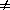Derivation of the Incomplete Dominance case of the General Selection Model

Given:    genotype:      AA          AB         BB
phenotype:      W0W1W2
or           1-s111-s2

Recall from the numerator of the General Selection Equation that

q[(q)(W2 - W1) + (p)(W1 - W0)]

So, to find q at equilibrium () (read as, "q hat") whereq = 0

set     0   = [(q)(1 - s2 - 1) + (p)(1 - 1 + s1)]

= -s2q + s1p

= s1(1 - q) - s2q

= s1 - s1q - s2q

Then    s1 = s1q + s2q = (q)(s1 + s2)

and=  (s1)/(s1 + s2)

Med6390 homework
: re-derive the above for "p" at equilibrium

Text material © 2004 by Steven M. Carr